# Codimension

(diff) ← Older revision | Latest revision (diff) | Newer revision → (diff)

The codimension (or quotient or factor dimension) of a subspaceof a vector spaceis the dimension of the quotient space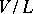; it is denoted by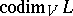, or simply by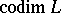, and is equal to the dimension of the orthogonal complement ofin. One has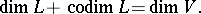Ifandare two subspaces ofof finite codimension, then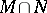and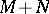are also of finite codimension, and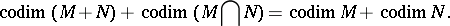The codimension of a submanifoldof a differentiable manifoldis the codimension of the tangent subspaceof the tangent space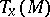at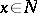. Ifandare finite-dimensional, then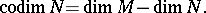Ifandare differentiable manifolds, ifis a submanifold ofand if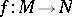is a differentiable mapping transversal to, thenThe codimension of an algebraic subvariety (or an analytic subspace)of an algebraic variety (analytic space)is the difference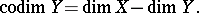How to Cite This Entry:
Codimension. Encyclopedia of Mathematics. URL: http://encyclopediaofmath.org/index.php?title=Codimension&oldid=18855
This article was adapted from an original article by V.E. GovorovA.F. Kharshiladze (originator), which appeared in Encyclopedia of Mathematics - ISBN 1402006098. See original article